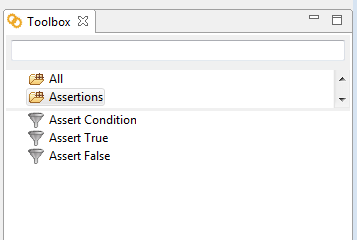“Validate Data with your own set of rules.”### Assert Condition

Asserts that an expression satisfies a certain condition. By assertion we mean that if the expression does not satisfies the condition, the operation and the task will be marked as an error.

Example: We want to assert that value of variable A is equal to value of variable B. This will be the condition to assert. Let’s assume that variable A=”A” and variable B =”B”. Therefore, the condition A (equal to) B will succeed but A(not equal to) B, A (less than) B or A (greater than) B, will fail.

#### Input parameters:

Expression - The name of the variable or an expression that you want to evaluate.
Operation - The type of comparison to perform. The options are: Equal To, Not Equal To, Less Than, Less Than or Equal To, Greater Than and Greater Than or Equal To.
Target Value - The value you want to compare against the other variable (or expression).

### Assert True

Asserts that a variable or an expression is true. By assertion we mean that if the expression is not true, the operation and the task will be marked as an error.

Example: We want to assert the following expression exampleValue==“10”. That will be the expression to assert is true. If exampleValue is not equal to “10” the operation and task will fail.

#### Input parameters:

Expression - The name of the variable (or an expression) that you want to assert is true.

### Assert False

Asserts that a variable or an expression is false. By assertion we mean that if the expression is not false, the operation and the task will be marked as an error.

Example: We want to assert the following expression exampleValue==“10”. That will be the expression to assert is false. If exampleValue is equal to “10” the operation and task will fail.

#### Input parameters:

Expression - The name of the variable (or an expression) that you want to assert is false.